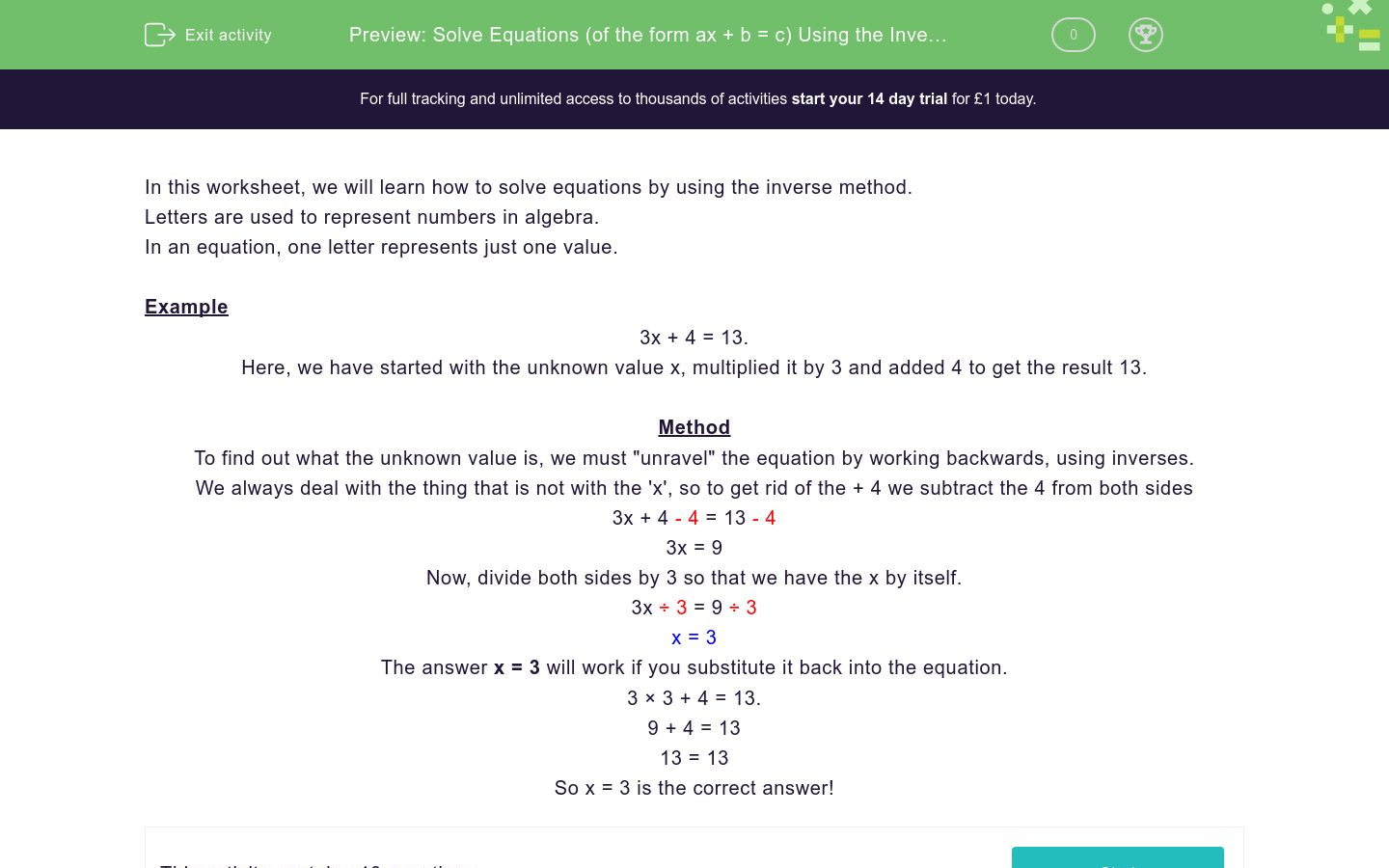# Solve Equations (of the form ax + b = c) Using the Inverse Method

In this worksheet, students will solve equations of the form ax + b = cusing the inverse method.Key stage:  KS 3

Curriculum topic:   Algebra

Curriculum subtopic:   Understand Expressions, Equations, Inequalities, Terms and Factors

Difficulty level:#### Worksheet Overview

In this worksheet, we will learn how to solve equations by using the inverse method.

Letters are used to represent numbers in algebra.

In an equation, one letter represents just one value.

Example

3x + 4 = 13.

Here, we have started with the unknown value x, multiplied it by 3 and added 4 to get the result 13.

Method

To find out what the unknown value is, we must "unravel" the equation by working backwards, using inverses.

We always deal with the thing that is not with the 'x', so to get rid of the + 4 we subtract the 4 from both sides

3x + 4 - 4 = 13 - 4

3x = 9

Now, divide both sides by 3 so that we have the x by itself.

3x ÷ 3 = 9 ÷ 3

x = 3

The answer x = 3 will work if you substitute it back into the equation.
3 × 3 + 4 = 13.

9 + 4 = 13

13 = 13

So x = 3 is the correct answer!

### What is EdPlace?

We're your National Curriculum aligned online education content provider helping each child succeed in English, maths and science from year 1 to GCSE. With an EdPlace account you’ll be able to track and measure progress, helping each child achieve their best. We build confidence and attainment by personalising each child’s learning at a level that suits them.

Get startedTry an activity or get started for free